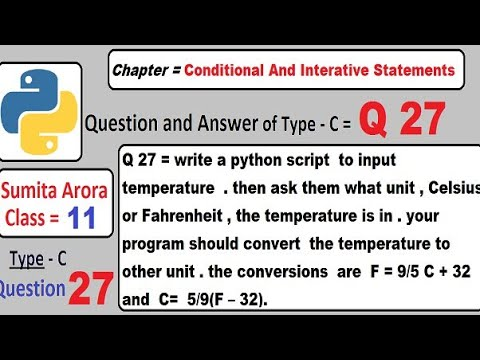## Q. Write a python script to input temperature. then ask them what unit, Celsius or Fahrenheit, the temperature is in. Your program should convert the temperature to other unit. the conversions are F = 9/5 C + 32 and C = 5/9(F – 32).

You can understand by Watching video :-```temp = float(input("Enter temperature = "))
unit = input("Enter unit of temperature (c or f) =")
if unit=="F" or unit == "f" :
c = (5/9)*(temp - 32)
print("Temperature in celsius = ",c,"C")
elif unit=="C" or unit == "c" :
f = (9/5)*temp + 32
print("Temperature in Fahrenheit = ",f,"F")
else :
print("Invalid unit ")
```

Output :-

Enter temperature = 37
Enter unit of temperature (c or f) =c
Temperature in Fahrenheit =  98.60000000000001 F

>>>

Enter temperature = 102
Enter unit of temperature (c or f) =f
Temperature in celsuis =  38.88888888888889 C

>>>

You can help us by Clicking on ads. ^_^
Please do not send spam comment : )

1.is the syntax correct?!

1.Yes, If you have doubt then please watch the video.